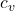## We’ve seen that for thermal radiation, the energy is of the form AVT4, where A is a universal constant, V is volume, and T is temperature. 1

Question

We’ve seen that for thermal radiation, the energy is of the form AVT4, where A is a universal constant, V is volume, and T is temperature. 1) The heat capacity CV also is proportional to a power of T, Tx. What is x

in progress 0
1 month 2021-08-07T20:09:18+00:00 1 Answers 1 views 0

this raise the temperature is x = 3

Explanation:

Heat capacity is the relationship between heat and temperature change

C = Q / ΔT

if the heat in the system is given by the change in energy and we carry this differential formulas= dE / dT

In this problem we are told that the energy of thermal radiation is

E = A V T⁴

Let’s look for the specific heat

c_{v} = AV 4 T³

the power to which this raise the temperature is x = 3